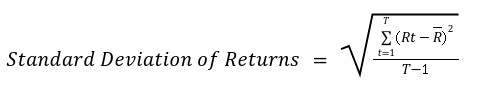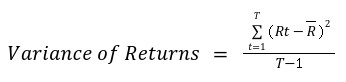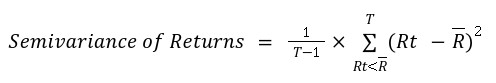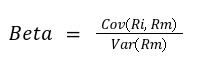# Low Volatility

Worried about market fluctuations? Consider the Low Volatility factor.

In the world of factor investing, avoiding volatility has become one of the most important investment considerations. Investing in equities with the lowest volatility is the essence of low volatility investing. But how is it beneficial? Analogy from the field of cricket will be examined now.

It goes without saying that the most interesting batsmen to watch are those who have the highest strike rates. However, the sad truth is that very few of these exciting batters are reliable scorers or have high career batting averages.

Similarly, the most thrilling stocks are frequently those that rise and fall extremely dramatically. But only a small percentage of these consistently perform over time and market circumstances. The low volatility aspect comes into play here.

It's one of the easier investment criteria to use because it can be determined quickly using only the stock price across time and relative to other stocks. Also, volatility characteristics of stocks  have been found to be consistent and slow to change.

## Low volatility

Low volatility stocks aren't the most interesting stocks to invest in. Their secret to making money isn't how quickly or far they rise, but how slowly they fall. They typically produce the best volatility adjusted returns, even if they don't always produce the best returns.As mentioned earlier, Low Volatility investing is construed as a defensive method aimed at protecting downside volatility during sharp market downturns.

This characteristic allows ‘Low Volatility’ securities to outperform the market by falling less during stressful periods and thereby generating positive excess returns. Several empirical studies have evidenced this phenomenon of Low Volatility stocks outperforming their high volatility counterparts.

Professors Thomas Merz, of Zurich University, and Pawel Janus, of VU University Amsterdam (Merz & Janus, 2016), conducted a study to evaluate the efficacy of the low volatility investing strategy. After analysing the data from 2000 through 2015 (a period that covers a market rally, the Global Financial Crisis (GFC) led recession, and post-GFC recovery), they conclude that low volatility enhanced portfolios exhibited extraordinary excess returns during the GFC. Their findings suggest that portfolios with exposure to the low volatility factor, on average, generated excess returns between 5.6% and 17.2% in the American market and 1.8% and 16.7% for the European market during corrections.

But how exactly is volatility defined by academics? Volatility, in the context of investments, is a statistical measure of the dispersion of returns generated by an asset. The most commonly used measure for this is the Standard Deviation of such returns. However, academicians and professionals use several other metrics to gauge the volatility of different securities. As such, the following measures have emerged as the most popular.

Standard Deviation of Returns
Variance of Returns
Semivariance of Returns
Beta of Securities

Standard Deviation of Returns

Standard deviation of returns is the simplest and most popular measure of volatility. It is a measure of the spread of the returns distribution exhibited by a security. Standard deviation indicates how far below or above the mean return do that security’s returns fall, on average. For instance, a standard deviation of 5% for a stock with an average return of 15% indicates that, on average, the stock’s returns would hover around 5% above or below the mean return of 15%.

Standard deviation, also known as sigma or 𝛔, can be calculated as:Where Rt refers the return of a security at time t (where t ranges from 1 to T) and R refers to the average security return over the entire period.

For instance, for a standard deviation calculated on monthly returns over 5 years, T = 60 (since there are a total of 60 monthly return observations), and Rt would range from R1 to R60, with each Rt indicating the return generated by the stock over that month. Finally, R would equal the average monthly return of the stock over the entire 60 monthly return observations.

Variance of Returns

Variance of returns is similar to standard deviation in the sense that the standard deviation is the square-root of the variance of returns. Although variance can be looked upon in the same way as standard deviation to gauge a security’s volatility, standard deviation standardizes the measure making it easily comparable. For instance, a stock with a variance of 2.25% and another with a variance of -2.25% will both have a standard deviation of 15%. This can make cross-sectional analysis problematic.

As mentioned above, since variance is the square of standard deviation of returns, the formula, shown below, is very intuitive and self-explanatoryWhere, Rt refers the return of a security at time t (where t ranges from 1 to T) and R refers to the average security return over the entire period.

Semivariance of Returns

Semivariance is yet another measure of dispersion, however, it is more suited as a measure of downside risk, rather than overall volatility. Unlike variance and standard deviation, that take into account both sides of the distribution of returns, the semivariance only takes into account the returns that fall below the average return over the period. Analysing semivariance of a portfolio can help avoid securities that pose high downside risks.

Since semivariance takes into account only the negative observations (i.e. those that fall below the mean returns), its computation is slightly different from variance and standard deviation.

Semivariance is computed as follows:Where, Rt refers the return of a security at time t (where t ranges from 1 to T) and R refers to the average security return over the entire period.

Here, Rt only includes returns over the entire period which fall below the mean or average return exhibited by the stock, unlike variance of returns which takes into account the upside as well as downside volatility of the returns.

Beta of Securities

Beta of a security indicates the sensitivity of that security’s returns to the returns on the market. It is a measure of the systematic risk inherent in the security i.e. risks that the asset/security is exposed to due overall market and economic conditions, which cannot be diversified away.

From a theoretical standpoint, the Beta of the entire market across all asset classes is equal to 1. However, since it is practically unmanageable to compute the Beta of all instruments in all asset classes and the fact that it has emerged as a popular measure only in the equity market, practitioners assign a Beta of 1 to the headline market indices (viz. Nifty 50, Sensex).

The Beta of a security is calculated as followsWhere,

Cov(Ri, Rm) is the covariance between the returns of the security and the returns on the index and

Var(Rm) refers to the variance of the returns of the index

It is clear from the formula above that the Beta of the index (against the index) is 1 since Cov(Ri, Rm) = Cov(Rm, Rm) = Var(Rm).

The higher the beta of a security, the greater its sensitivity to market returns. For instance, a stock with a Beta of 1.5 is expected to react to market movements a lot more than a stock with a Beta of 0.8. Also, the former would move more than the market while the latter would move less. It is also theoretically possible for securities to exhibit negative Beta, if their returns tend to move in a direction opposite to the index.

The definitions of volatility mentioned above are by no means exhaustive. Also, the choice of measure is related to the objective of the analysis as well as the characteristics of the underlying portfolio securities. Nevertheless, the Standard Deviation of returns remains the most widely-accepted measure of volatility within the investment community.